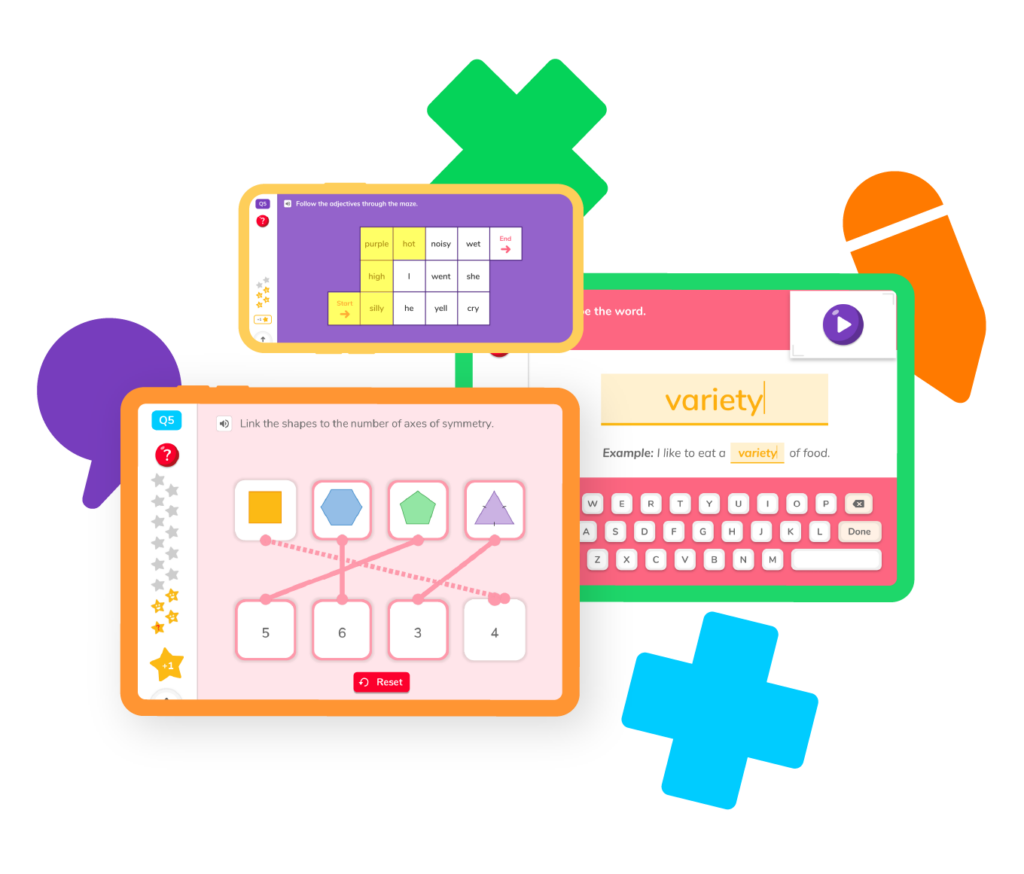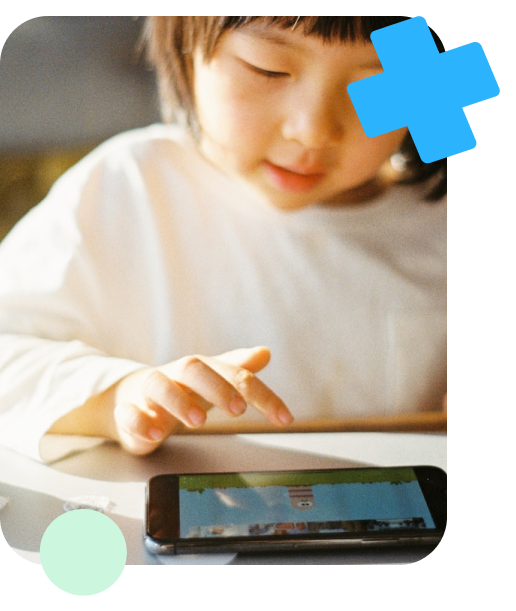The older students get, the more there is to learn! 3rd grade math presents exciting challenges like multiplication and division, graphing data, and more. DoodleMath has all of the guides, games, and practice problems your child needs to face the challenges of 3rd grade math and come out a champion.## Select a skillIt’s finally time to step into the exciting world of multiplication, division, and fractions! Whether you’re looking for more practice with a concept from class or trying to challenge your knowledge, DoodleMath has everything you need to become a real 3rd grade math wiz kid. Select a skill to practice or review.

## Multiplication

Learn how to multiply factors together to get a product.

## Division

Learn how to divide numbers and how division relates to multiplication.

## Word problems

Solve real-world word problems using multiplication and division.

## Fractions

Explore fractions as numbers and understand how to use them.

## Measurement

Learn and use standard units to determine the length of objects.

## Shapes

Use shapes and their attributes to solve for area, perimeter, and more.

## Multiplication

Learn how to multiply factors together to get a product.

## Division

Learn how to divide numbers and how division relates to multiplication.

## Word problems

Solve real-world word problems using multiplication and division.

## Fractions

Explore fractions as numbers and understand how to use them.

## Measurement

Learn and use standard units to determine the length of objects.

## Shapes

Use shapes and their attributes to solve for area, perimeter, and more.By the time they reach 3rd grade math, students have a better understanding of basic math concepts like addition and subtraction. Now, it’s time for them to branch into more complex mathematical ideas like multiplying, dividing, and using fractions as numbers.

Sometimes, making the leap can be a challenge. That’s why it’s so important to be prepared as a parent, so you can help your child become the best math student they can be!

Here’s what to expect in 3rd grade math. Keep in mind that standards vary by state, but most 3rd grade students are learning the same basic concepts.

### Operations and equations

1. Learn to multiply and divide up to 100. Students will learn their multiplication tables and how to multiply and divide to find products and dividends up to 100.
2. Understand the relationship between multiplication and division. They will understand that multiplication and division are inverse operations. For example, when you multiply one number by another number to get a product, you can also divide the product by one of those numbers to get the other number.
3. Solve two-step problems. Up until this point, students have usually been practicing one-step problems, like adding one number to another. This year, they will engage in two-step problems.

### Place value

1. Use place value to add and subtract. This means that students will start using concepts like subtraction with regrouping to solve math problems.
2. Understand how place value applies to multi-digit numbers. Essentially, 3rd graders will know that in the number 575, for example, there are five 100s, seven 10s, and five ones. Understanding this will help them add and subtract using strategies like borrowing.

### Fractions

1. Interpret fractions with denominators 2, 3, 4, 5, 6, 7 and 8 as parts of a whole. They will understand that 2/8 is equal to 1/4, and that it  represents 2 parts of an 8 part whole.
2. Compose and decompose fractions into equivalent fractions. 3rd grade math students will be able to simplify fractions as well as make them equal to each other.

### Measurement and data

1. Solve problems using measurement. They will be able to use their knowledge of units of measurement to solve real-life problems, such as how many minutes a person has to get ready if they wake up at a certain time and have to be out the door at a different time.
2. Understand area. 3rd graders will know that area is the measurement of a shape’s interior. They will also know how to calculate the area of certain shapes.
3. Understand perimeter. 3rd graders will understand that perimeter is the measure of a shape’s exterior. They will also know how to calculate the perimeter of shapes.
4. Represent and interpret data using charts and graphs. These won’t be the complicated charts and graphs they’ll encounter in middle school or high school, but students will begin to understand how to plot points and use charts and graphs to represent data.

### Geometry and shapes

1. Reason with shapes and their attributes. Students will build on their understanding of shapes by comparing similar shapes and describing what attributes they share and what makes them different.
2. Recognize and draw different types of polygons. Students will be able to successfully draw shapes like triangles, rhombuses, squares, rectangles, hexagons, and quadrilaterals.

Remember that all students learn at their own pace. If your student is struggling with concepts they’re learning in class, you can and should always talk to their teacher. DoodleMath helps kids build their confidence through fun, stress-free practice. Studies show that playing math games helps students learn and retain information!The answer to this question varies a little bit state-to-state, but the major concepts that students learn in 3rd grade math are multiplication and division. They learn not only how to multiply and divide, but how multiplication and division are related to each other.

3rd graders also learn about fractions as parts of a whole, how to graph and chart basic data, and how to solve multi-digit addition and subtraction problems.

They don’t learn algebra, necessarily, but they will begin to understand basic concepts that will help them later. For example, they might be given a problem that requires them to multiply an unknown number, X, with a known number to get a known product. They will then have to divide the product by the known number to find X.

The most important thing you can do is be there to support them when they need it. If they come to you for help, feel free to use our guides to refresh your math skills and help them succeed. If they request more support, like a math tutor, talk to their teacher about recommendations, or see if they might be willing to meet before or after school to help your child.

Not always, no. However, some studies suggest that 3rd grade is a difficult year for many students, since they are transitioning from “lower elementary school” to “upper elementary school.” This means they will be expected to learn more advanced concepts, like multiplication and division. Just be prepared for this transition and listen to your child. If they ask for additional support, subscribe to DoodleMath and supply them with endless guides, games, and practice problems to build their confidence.# Are you a parent, teacher or student?

Are you a parent or teacher?

## Hi there!

Book a chat with our team